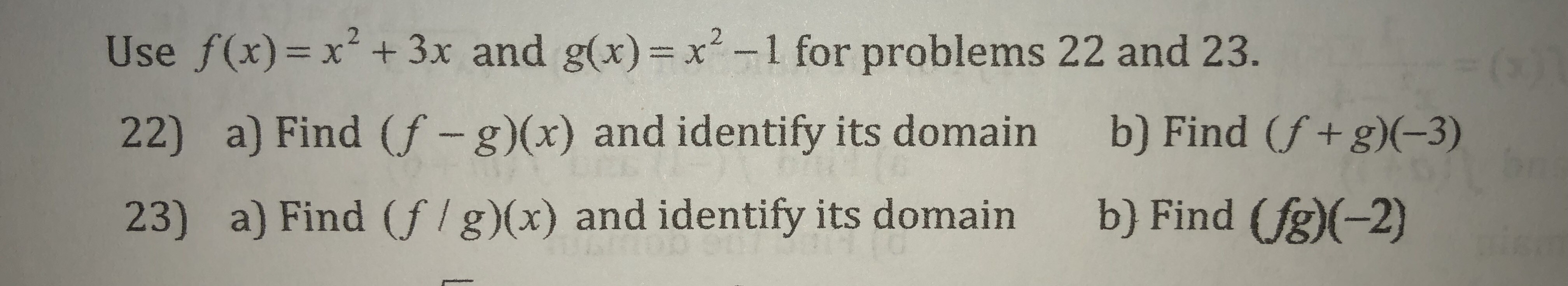# Use f (x)x2 +3x and gx)-1 for problems 22 and 23.22) a) Find (f - g)(x) and identify its domain b) Find (f+ g)(-3)23) a) Find (f/g)(x) and identify its domain b) Find (fg)(-2)

Question
23 viewshelp_outlineImage TranscriptioncloseUse f (x)x2 +3x and gx)-1 for problems 22 and 23. 22) a) Find (f - g)(x) and identify its domain b) Find (f+ g)(-3) 23) a) Find (f/g)(x) and identify its domain b) Find (fg)(-2) fullscreen
check_circle

Step 1

Note: Since the uploaded file has two problems and you haven't specified which one you need us to answer. We will answer the first problem (#22).

Step 2

Part(a)

We are given two functions f(x) and g(x) and we are supposed to find the function (f-g)(x) and its domain in this part.

In order to find (f-g)(x), we will use the formula (f-g)(x) = f(x) - g(x). So, basically, the function g(x) will be subtracted from function f(x) to write a formula for (f-g)(x) as shown below. We get (f-g)(x) = 3x+1 as shown below. Since this is a linear function, its domain will be all real numbers. We can write the domain in interval notation as (-inf, inf).

Step 3

Part(b)

In this part, we need to find the value of (f+g)(-3). In order to find this value, we will us...

### Want to see the full answer?

See Solution

#### Want to see this answer and more?

Solutions are written by subject experts who are available 24/7. Questions are typically answered within 1 hour.*

See Solution
*Response times may vary by subject and question.
Tagged in

### Algebra